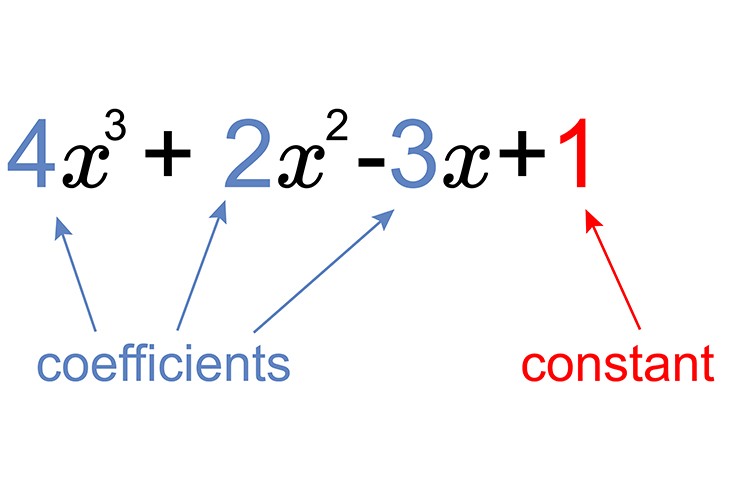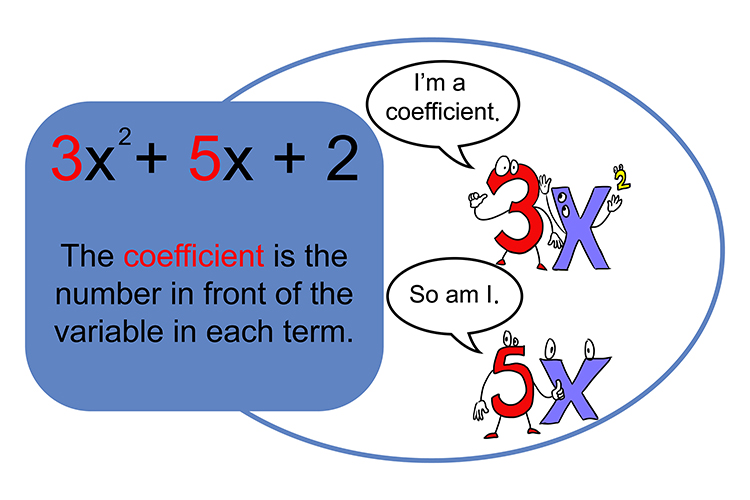# Coefficient

A number used to multiply a variable. Four is the coefficient in the term 4X.The company thought it would be a lot more efficient (coefficient) if everybody was called a number. The numbers were the internal office number.

Example 14 is the coefficient in the above equation.

Example 2Here we have three coefficients 4, 2 and -3.

Example 3